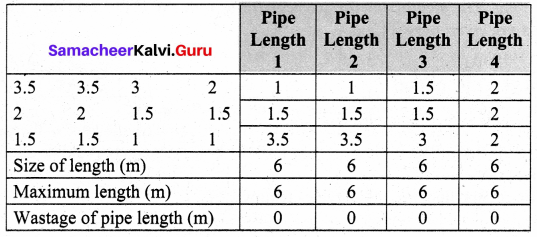Students can Download Maths Chapter 5 Information Processing Ex 5.2 Questions and Answers, Notes Pdf, Samacheer Kalvi 8th Maths Book Solutions Guide Pdf helps you to revise the complete Tamilnadu State Board New Syllabus and score more marks in your examinations.

## Tamilnadu Samacheer Kalvi 8th Maths Solutions Term 3 Chapter 5 Information Processing Ex 5.2

Question 1.
The sizes in MB, for nine computer files are given below. 53 82 61 38 23 41 16 34 42 The files are to be grouped into folders. Each folder may contain a maximum limit of 100 MB.
(a) Determine the minimum number of folders required.
(b) Use the first-fit method to group the files into folders.
(c) Use the first-fit decreasing method to group the files into folders.
Solution:
(a) Maximum number of folders required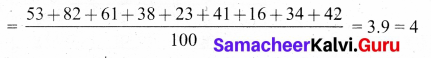(b) First fit method.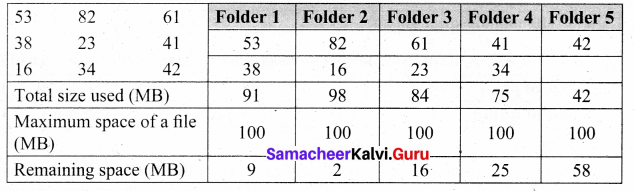(c) First fit decreasing method.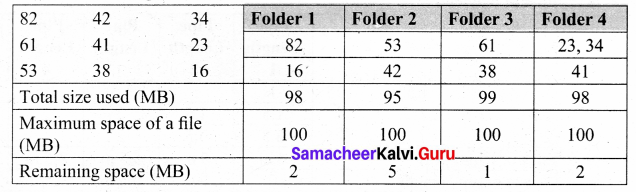Question 2.
A parcel delivery company has 4 motorcycles. Each motorcycle can carry a maximum load of 30kg. The weights of the parcels, (in kg), in the order they are waiting to be delivered are given below.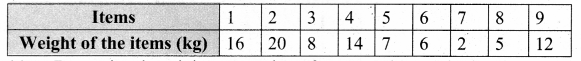(a) Determine the minimum number of motorcycles required .
(b) Use the first-fit method to show how the parcels could be allocated to the motorcycles.
(c) Use the first-fit decreasing method to show how the parcels could be allocated to the motorcycles.
(a) Minimum number of motorcycles required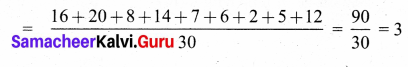(b) First fit method :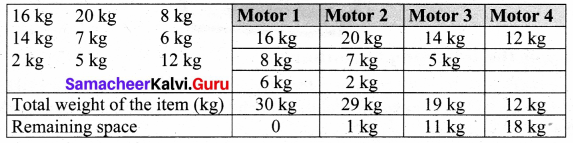(c) First fit decreasing method :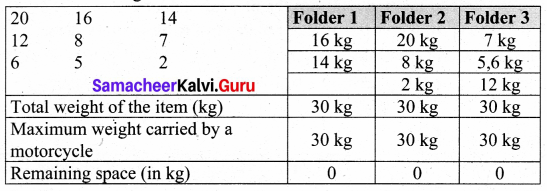Question 3.
A plumber wishes to cut the following sections from standard size of pipe each of length 6m.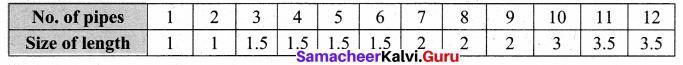Find the
(a) To cut according to the first-fit method calculate the wastage of the pipe length.
(b) To cut according to the first-fit decreasing method calculate the wastage of the pipe length,
Solution:
(a) First fit method :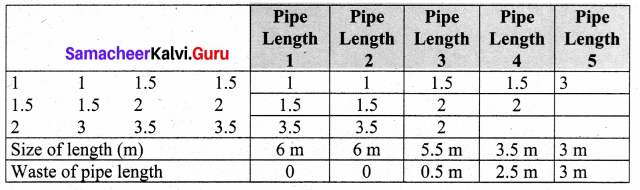(b) First fit decreasing method.